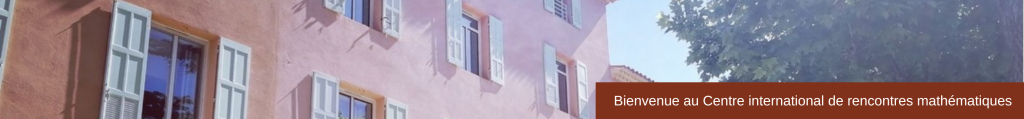CONFERENCE
 Spectrum of Random GraphsJanuary 4 – 8, 2016 The spectral analysis of matrices defined on deterministic or random graphs is a topic which attracts a growing attention in various branches of mathematics, computer science and theoretical physics. The motivation comes from many distinct directions: random matrices, random Schrödinger operators, quantum ergodicity, countable groups or expander graphs with an emphasis either on eigenvalues or eigenvectors. Scientific & Organizing Committee Charles Bordenave (Université Paul Sabatier, Toulouse)Alice Guionnet (MIT)Balint Virag (University of Toronto) Speakers Nalini Anantharaman (Université de Strasbourg) Quantum ergodicity on large graphs Agnes Backhausz (Alfréd Rényi Institute of Mathematics) Spectral measures of factor of i.i.d. processes on the regular tree Anna Ben-Hamou (Université Paris Diderot) A comparison of mixing times of the simple and non-backtracking random walks on random graphs with given degrees Jean-Marc Bouclet (Université Paul Sabatier, Toulouse) On some problems and techniques in spectral geometry Frédéric Faure (Université de Grenoble) Quantum chaos and random matrices Joel Friedman (University of British Columbia) Eigenvalues, Expected Traces, and Expected Zeta Functions of Random Graphs Ararat Harutyunyan (ENS Lyon) Connections between colorings in random graphs and digraphs Matthias Keller (University of Potsdam) Does diffusion determine the geometry of a graph? Antti Knowles (ETH Zurich) Local eigenvalues statistics for random regular graphs Marcin Kotowski (University of Toronto) Dyson’s spike in random Schrödinger operators Reimer Kuehn (Kings College London) Spectra of Random Stochastic Matrices and Relaxation in Complex Systems Can M. Le (University of Michigan) Concentration and regularization of random graphs Camille Male (Université Paris Descartes) Free probability and random graphs Laurent Massoulié (Inria Saclay-Ile de France) Non-backtracking spectrum of random graphs: community detection and non-regular Ramanujan graphs Pierre Mathieu (Aix-Marseille Université) Deviation inequalities and CLT for random walks on acylindrically hyperbolic groups Laurent Ménard (Université Paris Ouest Nanterre) Spectra of large diluted but bushy random graphs Shayan Oveis Gharan (University of Washington) Asymmetric Traveling Salesman Problem and Spectrally Thin Trees Eliott Paquette (Weizmann Institute of Science) The Poisson Voronoi tessellation in hyperbolic space Doron Puder (IAS, Princeton) Random irregular graphs are nearly Ramanujan Justin Salez (Université Paris Diderot) The cutoff phenomenon for random walk on random directed graphs Mira Shamis (Weizmann Institute) The Wegner orbital model Balázs Szegedy (Alfréd Rényi Institute of Mathematics) On the limit approach to random regular graphs Pierre Youssef (Université Paris Diderot) Invertibility of the adjacency matrix of a random